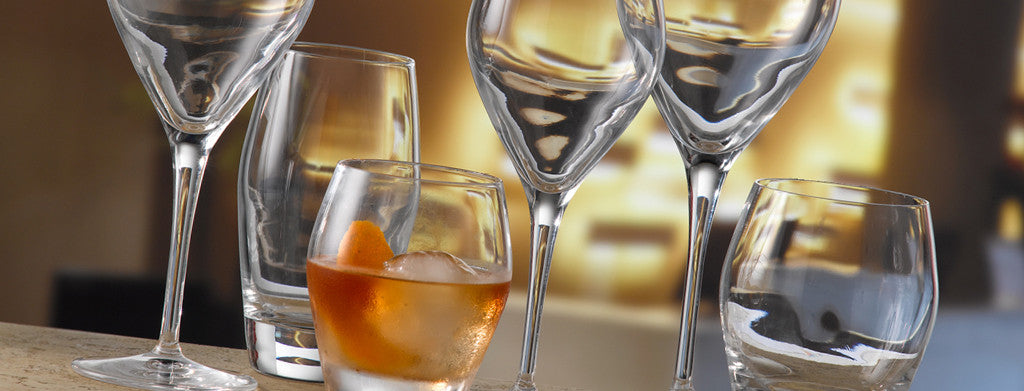Shopping Cart

### Refine

View all

#### Price# Glassware£2.03 each (ex VAT)£1.83 each (ex VAT)£1.72 each (ex VAT)£2.01 each (ex VAT)£1.77 each (ex VAT)£1.94 each (ex VAT)£1.84 each (ex VAT)£1.90 each (ex VAT)£1.85 each (ex VAT)£1.93 each (ex VAT)£1.72 each (ex VAT)£4.05 each (ex VAT)£1.93 each (ex VAT)£1.94 each (ex VAT)£1.84 each (ex VAT)£1.74 each (ex VAT)£1.70 each (ex VAT)£2.00 each (ex VAT)£24.97 each (ex VAT)£1.72 each (ex VAT)£2.02 each (ex VAT)£20.30 each (ex VAT)£22.67 each (ex VAT)£22.53 each (ex VAT)£1.98 each (ex VAT)£1.98 each (ex VAT)£3.66 each (ex VAT)£3.37 each (ex VAT)£3.92 each (ex VAT)£3.37 each (ex VAT)£3.92 each (ex VAT)£3.73 each (ex VAT)£1.86 each (ex VAT)£24.81 each (ex VAT)